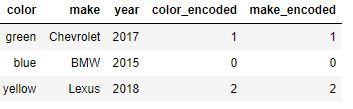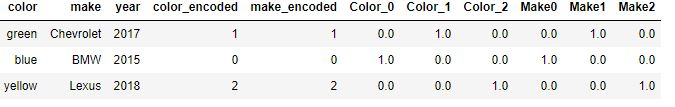Python One Hot Encoding with SciKit Learn

For machine learning algorithms to process categorical features, which can be in numerical or text form, they must be first transformed into a numerical representation. One widely used transformation in machine learning is called One-Hot Encoding. In the following article, I will show you how to implement One-Hot Encoding using SciKit Learn, a very popular python machine learning library.

After reading this post you will be able to:

• Use Scikit Learn to implement One-Hot Encoding
• Use the LabelEncoder Scikit learn class
• Use the OneHotEncoder Scikit learn class
• Use the LabelBinarizer Scikit learn class

Generate Test Data

In order to get started, let’s first generate a test data frame that we can play with.

import pandas as pd
#Create a test dataframe
df = pd.DataFrame([
['green', 'Chevrolet', 2017],
['blue', 'BMW', 2015],
['yellow', 'Lexus', 2018],
])
df.columns = ['color', 'make', 'year']

Our df variable contains a pandas dataframe with three rows and three columns about cars. The Color and Make columns are categorical features which will need to be transformed in order to use as inputs in the various machine learning algorithms. Let’s now perform one hot encoding on these two categorical variables.

OneHotEncoder

SciKit learn provides the OneHotEncoder class to convert numerical labels into a one hot encoded representation. This class requires numerical labels as inputs. Because our Color and Make columns contain text, we first need to convert them into numerical labels.  We will use SciKit learn labelencoder class to help us perform this step.

Start by initializing two label encoders, one for Color and one for Make. Next, call the fit transform method which will process our data and transform the text into one numerical value for each. Assign the results to 2 new columns, color_encoded and make_encoded.

from sklearn.preprocessing import LabelEncoder
le_color = LabelEncoder()
le_make = LabelEncoder()
df['color_encoded'] = le_color.fit_transform(df.color)
df['make_encoded'] = le_make.fit_transform(df.make)

You should now have the following dataframe:Looking at the color_encoded values: Green=1, Blue=0, Yellow=2. Similarly, look at our make feature and see how each has its own numerical value on the new make_encoded column.

Now that we have numerical values, we can utilize the OneHotEncoder class of SciKit Learn to perform one-hot encoding. Do so as below.

from sklearn.preprocessing import OneHotEncoder
color_ohe = OneHotEncoder()
make_ohe = OneHotEncoder()
X = color_ohe.fit_transform(df.color_encoded.values.reshape(-1,1)).toarray()
Xm = make_ohe.fit_transform(df.make_encoded.values.reshape(-1,1)).toarray()

First, initialize the OneHotEncoder class to transform the color feature. The fit_transform method expects a 2D array, reshape to transform from 1D to a 2D array.

The fit_transform method returns a sparse array. Use the toarray() method to return a numpy array and assign this to variable X which has our one hot encoded results.

To add this back into the original dataframe you could do as below.

dfOneHot = pd.DataFrame(X, columns = ["Color_"+str(int(i)) for i in range(X.shape)])
df = pd.concat([df, dfOneHot], axis=1)

dfOneHot = pd.DataFrame(Xm, columns = ["Make"+str(int(i)) for i in range(X.shape)])
df = pd.concat([df, dfOneHot], axis=1)

The end result is shown below. We added back the one hot encoded values into our original data frame for inspection. We now have 3 new dummy features for color and 3 for make and could use these as inputs into our machine learning models.The above was a two-step process involving the LabelEncoder and then the OneHotEncoder class. SciKit learn provides another class which performs these two-step process in a single step called the Label Binarizer class.

Label Binarizer

SciKit learn provides the label binarizer class to perform one hot encoding in a single step. The below code will perform one hot encoding on our Color and Make variable using this class.

from sklearn.preprocessing import LabelBinarizer
color_lb = LabelBinarizer()
make_lb = LabelBinarizer()
X = color_lb.fit_transform(df.color.values)
Xm = make_lb.fit_transform(df.make.values)

In an easy single step process X and Xm contains the one-hot encoded numpy array for the color and make features. X returns the below array:

array([[0, 1, 0],
[1, 0, 0],
[0, 0, 1]])

To convert from the one-hot encoded vector back into the original text category, the label binarizer class provides the inverse transform function. This function takes as inputs a numpy array or sparse matrix with shape [n_samples, n_classes] and returns the original text values.

For example, the first value in our X array contains the one-hot encoded vector for the color green. If we pass this into the inverse transform function, it will return green as shown below.

green_ohe = X[]
color_lb.inverse_transform(green_ohe)

Returns the green label: array(['green'], dtype='<U6')

Conclusion

We have seen two methods to implement one-hot encoding using scikit learn.

The first involved a two-step process by first converting color and make features into a numerical label using the label encoder class. With numerical labels, we then utilize the one-hot encoder class.

The second method involves a one-shot process to implement one-hot encoding in a single step using the label binarizer class. We also saw how to go backward, from the one-hot encoded representation into the original text form

There are other ways to implement one-hot encoding in python such as with Pandas data frames.

You can read more about One-Hot Encoding and its Pandas implementation in the post: One-Hot encoding with Pandas made Simple.

To continue learning, about one hot encoding and other machine learning techniques I highly recommend the following book:Disclosure: The link above is an affiliate link, meaning, I will earn a commission if you click through and make a purchase.